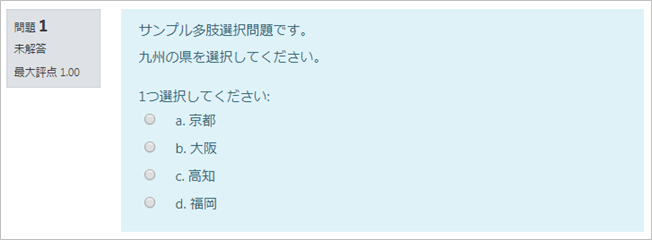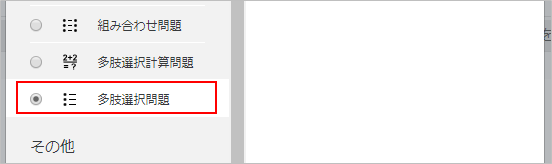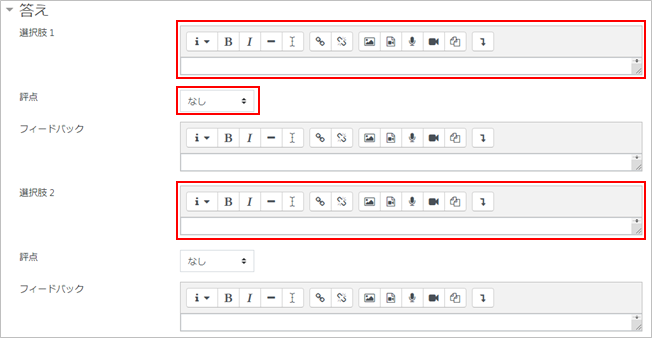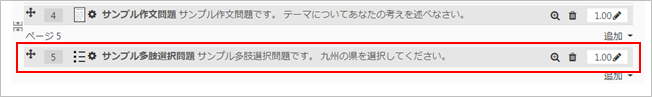#### A multiple choice question have one or multiple correct answers. In the case of multiple answers, there is a partial point system where points can be assigned to each choice. If you want to get an exact answer, use “All or nothing multiple choice question type”.

Refer to:
10-01-20.How to use quiz sample questions in your course1. Choose [Multiple choice] from the list of question type to add and click [Add].2. Enter “Question name” and “Question text.” Also enter “Default mark.”3. Set “One or multiple answers?”
If [Shuffle the choices?] is enabled, the order of the choices is randomly shuffled.
“With Number the choices?,” you can choose from numbers and alphabets.For Multiple Answers: The correct answer should be assigned a point total of 100% (50% for each correct answer). Incorrect answers should either be scored so that the point percentages sum to -100% (or -33.33333% for each of the three incorrect answers) or all of them should be set to -100%.
Note: A negative sum of the percentages will not result in a score less than zero.5. Click on [Save changes], and now multiple choice question is added to the quiz.6. Check the question on the preview display.Last Updated on 2021-03-30